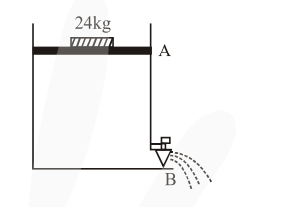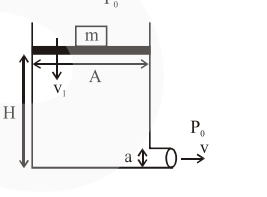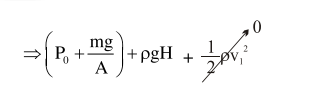# Consider a water tank as shown in the figure.`
Question:

Consider a water tank as shown in the figure. It's cross-sectional area is $0.4 \mathrm{~m}^{2}$. The tank has an opening $B$ near the bottom whose crosssection area is $1 \mathrm{~cm}^{2}$. A load of $24 \mathrm{~kg}$ is applied on the water at the top when the height of the water level is $40 \mathrm{~cm}$ above the bottom, the velocity of water coming out the opening $B$ is $\mathrm{v} \mathrm{ms}^{-1}$. The value of $\mathrm{v}$, to the nearest integer, is____________. [Take value of $g$ to be $10 \mathrm{~ms}^{-2}$ ]Solution:$\mathrm{m}=24 \mathrm{~kg}$

$\mathrm{~A}=0.4 \mathrm{~m}^{2}$

$\mathrm{a}=1 \mathrm{~cm}^{2}$

$\mathrm{H}=40 \mathrm{~cm}$

Using Bernoulli's equation$=\mathrm{P}_{0}+0+\frac{1}{2} \rho \mathrm{v}^{2}$ .......(1)

$\Rightarrow$ Neglecting $\mathrm{v}_{1}$

$\Rightarrow \mathrm{v}=\sqrt{2 \mathrm{gH}+\frac{2 \mathrm{mg}}{\mathrm{A} \rho}}$

$\Rightarrow \mathrm{v}=\sqrt{8+1.2}$

$\Rightarrow \mathrm{v}=3.033 \mathrm{~m} / \mathrm{s}$

$\Rightarrow \mathrm{v} \simeq 3 \mathrm{~m} / \mathrm{s}$